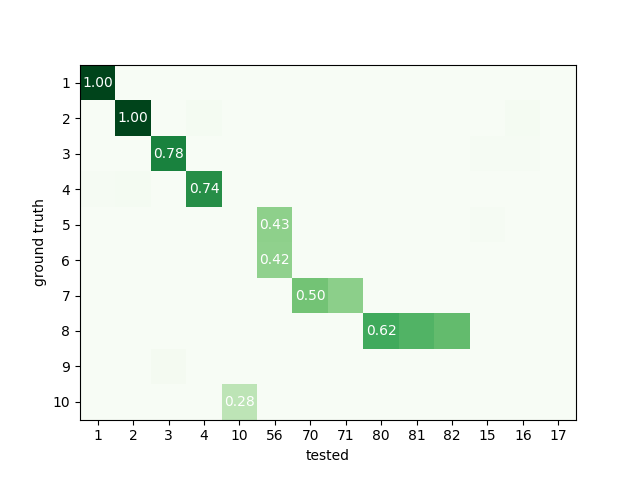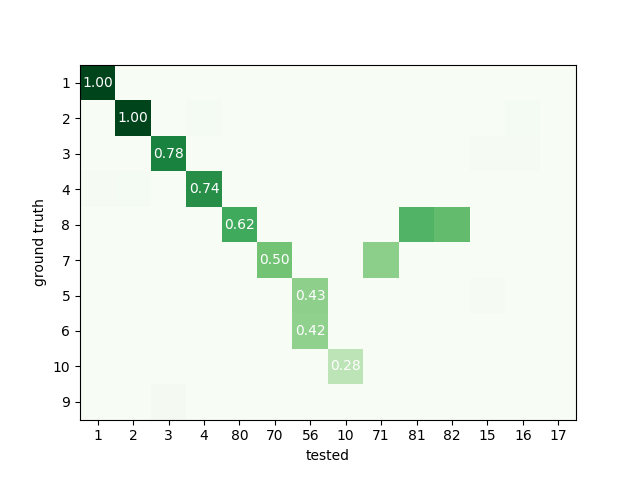# Explore sorters weaknesses with with ground-truth comparison¶

Here a synthetic dataset will demonstrate some weaknesses.

Standard weaknesses :

• not all units are detected

• a unit is detected, but not all of its spikes (false negatives)

• a unit is detected, but it detects too many spikes (false positives)

Other weaknesses:

• detect too many units (false positive units)

• detect units twice (or more) (redundant units = oversplit units)

• several units are merged into one units (overmerged units)

To demonstrate this the script generate_erroneous_sorting.py generate a ground truth sorting with 10 units. We duplicate the results and modify it a bit to inject some “errors”:

• unit 1 2 are perfect

• unit 3 4 have medium agreement

• unit 5 6 are over merge

• unit 7 is over split in 2 part

• unit 8 is redundant 3 times

• unit 9 is missing

• unit 10 have low agreement

• some units in tested do not exist at all in GT (15, 16, 17)

Import

```import numpy as np
import pandas as pd
import matplotlib.pyplot as plt
import seaborn as sns

import spikeinterface.extractors as se
import spikeinterface.sorters as ss
import spikeinterface.comparison as sc
import spikeinterface.widgets as sw

from generate_erroneous_sorting import generate_erroneous_sorting
```

Out:

```/home/docs/checkouts/readthedocs.org/user_builds/spikeinterface/conda/latest/lib/python3.8/site-packages/seaborn/cm.py:1582: UserWarning: Trying to register the cmap 'rocket' which already exists.
mpl_cm.register_cmap(_name, _cmap)
mpl_cm.register_cmap(_name + "_r", _cmap_r)
mpl_cm.register_cmap(_name, _cmap)
mpl_cm.register_cmap(_name + "_r", _cmap_r)
mpl_cm.register_cmap(_name, _cmap)
mpl_cm.register_cmap(_name + "_r", _cmap_r)
mpl_cm.register_cmap(_name, _cmap)
mpl_cm.register_cmap(_name + "_r", _cmap_r)
mpl_cm.register_cmap(_name, _cmap)
mpl_cm.register_cmap(_name + "_r", _cmap_r)
mpl_cm.register_cmap(_name, _cmap)
mpl_cm.register_cmap(_name + "_r", _cmap_r)
```

Here the agreement matrix

```sorting_true, sorting_err = generate_erroneous_sorting()
comp = sc.compare_sorter_to_ground_truth(sorting_true, sorting_err, exhaustive_gt=True)
sw.plot_agreement_matrix(comp, ordered=False)
```Out:

```<spikeinterface.widgets.agreementmatrix.AgreementMatrixWidget object at 0x7f59c8f2d8b0>
```

Here the same matrix but ordered  It is now quite trivial to check that fake injected errors are enlighted here.

```sw.plot_agreement_matrix(comp, ordered=True)
```Out:

```<spikeinterface.widgets.agreementmatrix.AgreementMatrixWidget object at 0x7f59c2d4d400>
```

Here we can see that only Units 1 2 and 3 are well detected with ‘accuracy’>0.75

```print('well_detected', comp.get_well_detected_units(well_detected_score=0.75))
```

Out:

```well_detected [1, 2, 3]
```

Here we can explore “false positive units” units that do not exists in ground truth

```print('false_positive', comp.get_false_positive_units(redundant_score=0.2))
```

Out:

```false_positive [15, 16, 17]
```

Here we can explore “redundant units” units that do not exists in ground truth

```print('redundant', comp.get_redundant_units(redundant_score=0.2))
```

Out:

```redundant [71, 81]
```

Here we can explore “overmerged units” units that do not exists in ground truth

```print('overmerged', comp.get_overmerged_units(overmerged_score=0.2))
```

Out:

```overmerged 
```

Here we can explore “bad units” units that a mixed a several possible errors.

```print('bad', comp.get_bad_units())
```

Out:

```bad [10, 56, 71, 81, 15, 16, 17]
```

There is a convenient function to summary everything.

```comp.print_summary(well_detected_score=0.75, redundant_score=0.2, overmerged_score=0.2)

plt.show()
```

Out:

```SUMMARY
-------
GT num_units: 10
TESTED num_units: 13
num_well_detected: 3
num_redundant: 2
num_overmerged: 1
num_false_positive_units 3